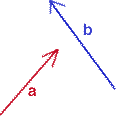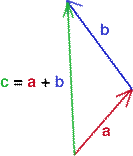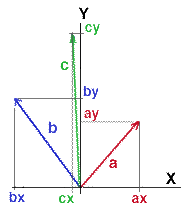Introduction

Vectors are entities that have direction and length ie. magnitude. Typically, they are defined by 2 or 3 values. Part of the confusion in dealing with vectors is the way they are represented in illustrations. Often they are represented as free vectors that can be moved into arbitary locations depending on the concept that is being explained.

However, when it comes to performing certain mathematical operations on vectors it is essential to be aware that vectors are not "free" but are fixed at the origin of a coordinate system. For example, although it makes visual sense to show a surface normal (a type of vector) extending directly from, say a polygon, the normal is, in fact, not located at the surface of the "its" polygon but is located at the origin of the coordinate system in which the polygon is defined and as such must be treated as fixed vectors.Figure 1Figure 2

 Figure 1 shows two free vectors, a and b drawn in arbitary locations. In figure 2, for the purpose of showing what is meant by vector addition the vector labeled b has been moved so that its "tail" coincides with the "head" of the vector a. Using free vectors in this way makes it clear that the overall effect of travelling, first, along vector a, then along vector b, is the same as taking the "short-cut" along vector c.This is what is meant by the notation,     c = a + b where a, b and c are vectors.

Fixed Vectors

When considering certain mathematical operations on vectors it is essential to be aware that vectors are not "free" but are fixed at the origin of a coordinate system. For example, although it makes visual sense to show a surface normal (a type of vector) extending directly from, say a polygon, the normal is not located at the surface of the polygon but is located at the origin of the coordinate system in which the polygon is defined and as such the vector must be treated as fixed vector.

Fixed vectors cannot join themselves "tail" to "head". When two vectors, say a and b, are added together (figure 3) the result is a third vector. To calculate the components of the third vector, the corresponding components of a and b are added together ie.

cx = ax + bx
cy = ay + by